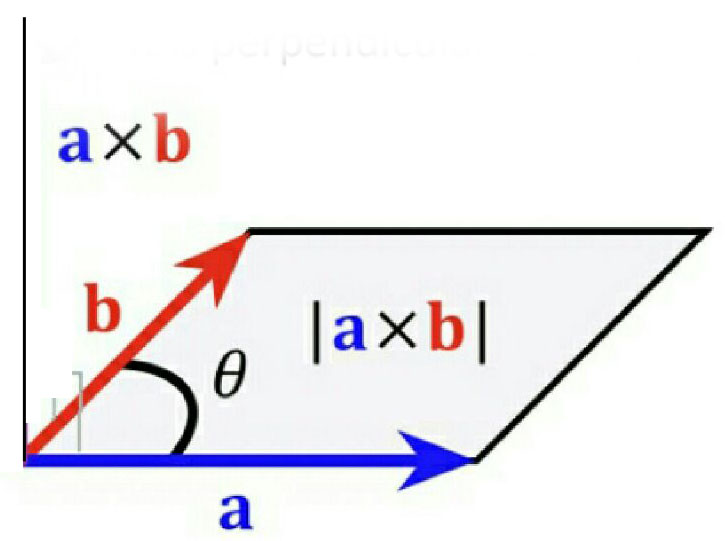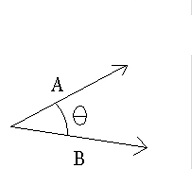# Vectors  are such that . Then the vector parallel to  is Option 1) Option 2) Option 3) Option 4)

As learnt in

Vector or cross product -

Vector or cross product of two vector & written as is a single vector whose magnitude is equal to product of magnitude of & and the sine of smaller angle  between them.

- whereinFigure 6 shows representation of vector or cross product of vectors.

shows representation of vector or cross product of vectors

and

Scalar , Dot or Inner Product -

Scalar product of two vector & written as is a scalar quantity given by the product of magnitude of & and the cosine of smaller angle between them.

- whereinshowing representation of scalar products of vectors.

Option 1)

This option is incorrect

Option 2)

This option is incorrect

Option 3)

This option is incorrect

Option 4)

This option is correct

Exams
Articles
Questions